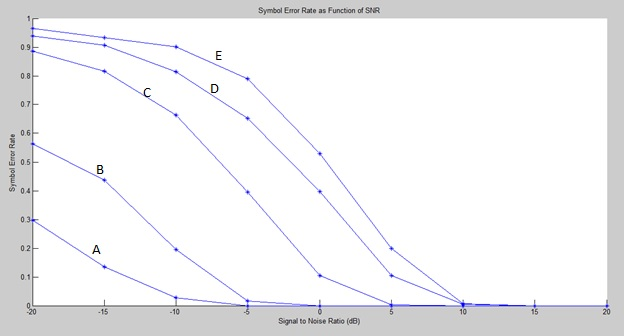# Why showing different number of bits in different modulations?

Why do different modulation schemes(BPSK, QPSK, QAM16, QAM64 and StarQAM16) yield different numbers of receive bits at high SNRs? For some SNRs, lower order modulation schemes yield higher bit rates. Why is this the case?1 Like

hi Tharinduhigher order modulation gives higher data rate because every modulation symbol carries diff number of bits which increase with higher order of modulation for example 16QAM means 16 symbol so we need log(16)=4 bits to map each symbol and identify each one among the other 64QAM gives log 64 = 6 bit per symbol so if SNR is high whish increases the error so this decreases no of bits received correctly (BER is function of Eb/N0) hence to mitigate the error we adding redundancy bits(error detection and correction bits) in the transmitted data block of fixed size of number of bits to get the receiver detect the error and correct it when error occurs and this techniques is called forward error correction by convolution encoder(low rate and signaling ) and turbo encoder(higher rate )
practically we use lower modulation order such as BPSK IN signaling to reduce error

1 Like